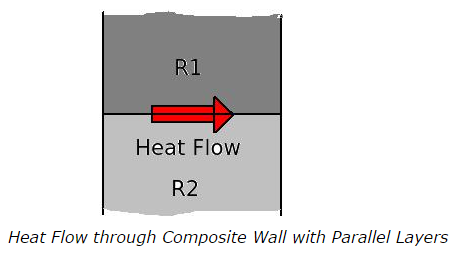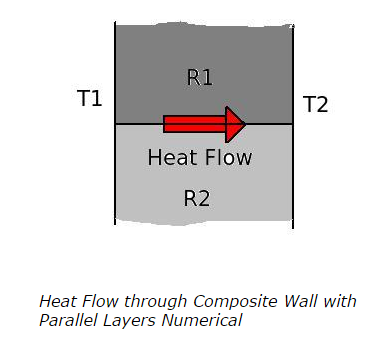# Composite Wall - Parallel & Series Heat Flow Path - Steady-State Conduction

## Composite Wall - Parallel & Series Heat Flow Path - Steady-State Conduction - MCQs with Answers

Q1. Consider a composite wall with two layers combined in parallel and heat is transferred through wall as shown in figure. The thermal resistances of two layers are R1 and R2 respectively. How is the total thermal resistance (R) of the wall calculated?a. R = (R1 + R2) / R1R2
b. R = R1R2 / (R1 + R2)
c. R = R1 / (R1 + R2)
d. R = R2 / (R1 + R2)

ANSWER: b. R = R1R2 / (R1 + R2)

Q2. When the layers of a composite wall are in series and the thermal resistance of every individual layer is given then the total thermal resistance of the composite wall is given by

a. substantiating lower value thermal resistance from higher value thermal resistance
b. adding both the thermal resistances
c. multiplying both the thermal resistances
d. none of the above

Q3. A composite wall of two layers in parallel as shown in figure has the rate of heat transfer 2 kW/m2. The thermal resistance R1 = 0.6 K/W and R2 = 0.4 K/W. The initial temperature T1 is at 1000 K. What is the temperature T2 of the other surface?a. 320 K
b. 520 K
c. 220 K
d. insufficient data